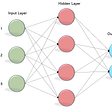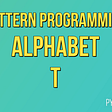# Machine Learning Interview Questions And Answers

https://www.blog.duomly.com/how-to-pass-machine-learning-interview/

Machine learning (ML) is a rising field. It offers many interesting and well-paid jobs and opportunities. To start working in machine learning, you should become familiar with:

mathematical fundamentals — linear algebra, calculus, optimization, probability, and statistics, etc.,

• machine learning fundamentals — prepare data, validate and improve results, interpret results, recognize and avoid overfitting, etc.,
• often used ML algorithms and methods — linear regression, decision trees, support vector machines, k nearest neighbors, neural networks, k-means clustering, principal component analysis and more,
• programming — some knowledge of Python and/or R is desirable, as well as the ability to use the libraries for machine learning (like NumPy, Pandas, scikit-learn, Matplotlib, Tensorflow and more), etc.

Each of these and some other items might be touched in an ML interview. There is a large number of possible questions and topics.

This article presents 12 general questions (with the brief answers) appropriate mainly for beginners and intermediates. These questions are not related to any particular machine learning algorithm or method. They are about some of the fundamental machine learning topics.

1. What are the types of machine learning algorithms?

There are three main types of ML algorithms:

• Supervised learning — modeling mathematical dependencies (mappings) between given input data (features) and output data. It mainly solves the regression and classification problems. Regression problems have continuous and numerical outputs, while classification deals with discrete, often categorical outputs.
• Unsupervised learning — finding structures, rules, and patterns among input data without any outputs provided. There are several categories of unsupervised learning methods, such as cluster analysis, association rule learning, anomaly detection, etc.
• Reinforcement learning — taking actions that maximize a reward, as well as learning and improving continually based on past experience.

There is also the semi-supervised learning. It is between supervised and unsupervised learning.

2. What are data standardization and normalization?

Standardizing datasets in ML enables comparing the features with different units and is a requirement for many ML methods (like support vector machines, neural networks, k-means clustering, linear discriminant analysis and many more).

Standardization usually means that the features are rescaled so that they have the mean of zero and the standard deviation of one.

In some cases, one can use the min-max normalization instead. It rescales the features so that the minimum value is mapped to zero, maximal value to one, while all other values are linearly distributed between zero and one.

3. What is R2?

R2 or the coefficient of determination is a numerical value that represents the variation in outputs that can be explained by the inputs. It is used as a measure of the goodness of fit, i.e. how close are the actual and predicted outputs in regression problems. Larger values are better and R2 = 1 means the perfect fit.

4. Explain type I and type II errors

A type I error (false positive error) represents the incorrect rejection of a true null hypothesis. A type II error (false negative error) is the incorrect acceptance of a false null hypothesis. (A positive result is related to the rejection of a null hypothesis.)

5. Explain conditional probability

Conditional probability is the probability that some event will occur given that some other event has occurred. The probability that the event E will occur given that the event F has occurred is: P(E|F) = P(EF) / P(F), where P(EF) is the probability that both events will occur, while P(F) is the probability that F will occur.

6. What are training, validation and test datasets?

The training set is the part of the dataset applied to train the model, i.e. to fit its parameters. The validation set is another part of the dataset used during hyper-parameters tuning. Finally, the test set is the third part of the dataset for evaluation of the performance of the chosen model. These three parts of the datasets are usually independent and chosen randomly.

7. What is overfitting?

Overfitting occurs when a model learns existing data to well. In such cases, it learns both the existing dependencies among data, but also random fluctuations (noise).

Overfitted models usually perform well on training data, but poorly when applied to unseen (test) data.

Complex or flexible models are more prone to overfitting.

8. What is dimensionality reduction?

Dimensionality reduction is a set of techniques to decrease the number of features (input variables) of a machine learning model. There are two main approaches to dimensionality reduction:

• feature selection — selecting a subset of the most important features,
• feature extraction — replace all features with a new, smaller set of derived features that minimize redundancy.

9. What is the kernel trick?

The kernel trick is related to mapping data into a higher-dimensional space to make it clearly separable. It avoids computing the new coordinates of the data points in this space. The kernel trick is important for support vector machines and principal component analysis.

10. Explain the gradient descent method

Gradient descent is a fast, iterative, approximate, gradient-based optimization method that aims to find a local minimum of a function. It moves iteratively from the starting point in the direction of the steepest descent. The direction and step are calculated using the negative gradient of the function.

If the function is convex, gradient descent searches for the global minimum.

11. What is clustering?

Clustering or cluster analysis is a process of grouping data points (observations) into two or more groups (clusters) based on the similarities among their features. Similar points should be in the same group.

Some of the clustering methods are k-means clustering, mean-shift clustering, agglomerative clustering, spectral clustering, affinity propagation, DBSCAN, etc.

The bias is the difference between the outputs predicted by the model and actual outputs. The variance is the measure of the variability of the model predictions for different training sets. Simple models might be underfitted and have high bias and low variance. Contrary, complex models (that have many parameters) sometimes suffer from overfitting having a low bias and high variance. What we want are the lowest possible values of both bias and variance. To accomplish this, we have to find a model of the appropriate complexity.

Of course, job interviews are not only about asking and answering field-related questions. You should follow also some of the general recommendations for preparing for job interviews like:

• making a good research of the company,
• being ready to explain your experience in the field, your interests, and the reasons why do you want the job,
• being able to emphasize your strengths and explain why you are a good candidate for the job,
• dressing and behaving appropriately,
• asking smart questions about the role of interest and the company, etc.

The article was prepared by our teammate Mirko.

--

--

--

## More from Duomly - Programming courses online

www.duomly.com - We believe everyone can learn how to code, so we are making learning fun and easy!

## Multi layer Perceptron (MLP) Models on Real World Banking Data## The Power of Data — A Review of MLconf 2019## Retirement of convolutions## Sentiment analysis in stock market## RoIMix: Proposal-Fusion among Multiple Images for Underwater Object Detection 論文閱讀筆記## Face Recognition DCNNCore developer in www.duomly.com - Programming online courses

## How to Print Alphabet T in Python?## Different Ways to Solve Anagrams in Python## Building fast k-means from scratch## NFL Twitter Sentiment: Vikings at Packers, Week 17, 2021